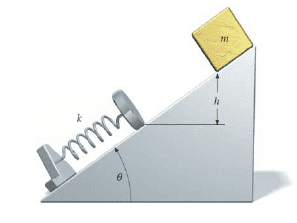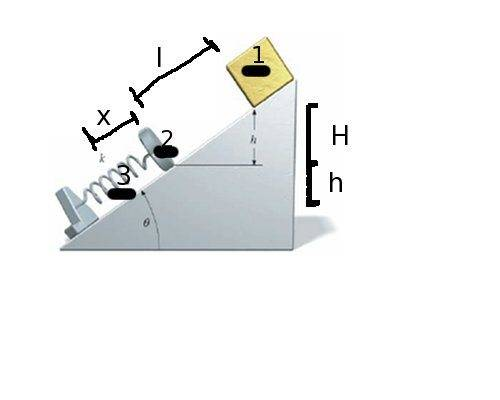# Conservation Of Energy, Block Sliding Down Incline Onto Spring.

Creepypunguy

## Homework StatementA block of mass m starts from rest at a height h and slides down a frictionless plane inclined at angle θ with the horizontal, as shown below. The block strikes a spring of force constant k.
Find the distance the spring is compressed when the block momentarily stops. (Let the distance the block slides before striking the spring be l. Use the following as necessary: m, θ, k, l, and g.)

## Homework Equations

Conservation of Energy:
GPE : U = mgh
K = (1/2)mv2
Spring = (1/2)kx2

## The Attempt at a Solution

My Diagram:Let H = l*sinθ
Let h = x*sinθ

Moment 1: E = mg(H+h) = mgsinθ(l+ x)

Moment 2: E = (1/2)mv2 + mgh = (1/2)mv2 + mgx*sinθ

Moment 3: E = (1/2)kx2

-------- Attempts to get rid of x2 led to
x = h/sin
(1/2)k(h/sinθ)2 = mgsinθ(l+ x) ;;;
(kh2/2sin2θ) = mgsinθ(l+ x) ;;;
(kh2/2) = mglsin3θ + mgxsin3θ ;;;
(kh2/2) - mglsin3θ = mgxsin3θ ;;;

x = k*h2/2mgsin3θ - l

Mentor
Moment 1: E = mg(H+h) = mgsinθ(l+ x)

Moment 2: E = (1/2)mv2 + mgh = (1/2)mv2 + mgx*sinθ

Moment 3: E = (1/2)kx2
This is good.

-------- Attempts to get rid of x2 led to
x = h/sin
(1/2)k(h/sinθ)2 = mgsinθ(l+ x) ;;;
(kh2/2sin2θ) = mgsinθ(l+ x) ;;;
(kh2/2) = mglsin3θ + mgxsin3θ ;;;
(kh2/2) - mglsin3θ = mgxsin3θ ;;;

x = k*h2/2mgsin3θ - l
Don't try to express things in terms of "h"--that's an unknown directly related to "x".

Hint: Just compare moments 1 and 3. Solve that equation!

Homework Helper
As h=xsinθ, your last equation does not give x explicitly in terms of the known quantities, k, m, θ, L, and g.

ehild

Creepypunguy
So by solving equations 1 and 3 I got
mglsinθ+mgxsinθ = (1/2)kx2
0 = (k/2)x2 - mgsinθx - mglsinθ